What’s the Multiplication Area Model and How Do You Teach it? Comprehensive Guide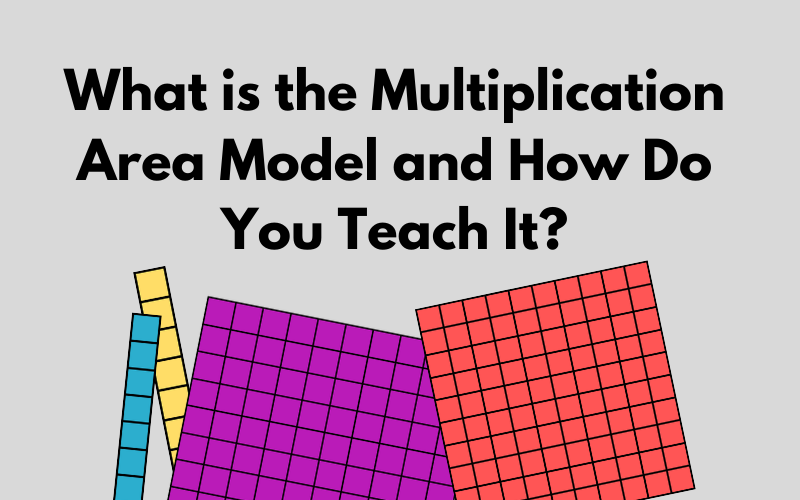The area model is a strategy for multiplication. Most often we see this as a strategy for multi-digit multiplication. It is an excellent way for students to work with multiplication in a more conceptual way before transitioning to the traditional algorithm. First of all, let’s take a look at how the area model is typically used.

The Area Model: What We Typically See

The area model is typically seen like this for a 2-digit by 2-digit multiplication problem. The factors become the side lengths of our rectangle. The area of the rectangle will be our product.

We decompose the factors into tens and ones. Then we multiply the parts to find the product for each part of our rectangle. In this example, we multiplied 10×10 to make 100, 10×3 to make 30, 2×10 to make 20, and 2×3 to make 6. Then we add up all four of these “partial products” – 100+30+20+6=156. Therefore our product (or the total area of the rectangle) is 156.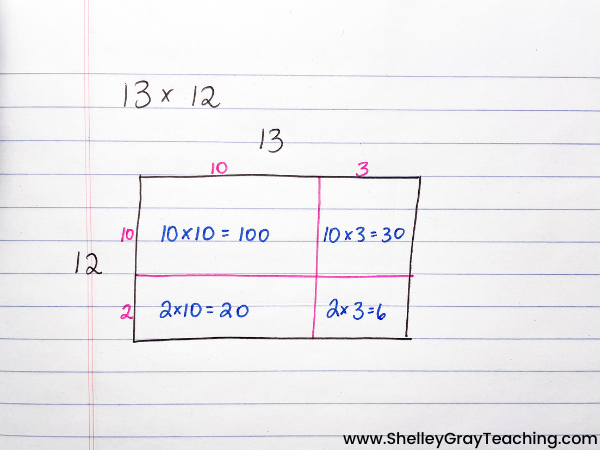Here’s an example of how we can use the area model to solve different types of problems with other factors.But isn’t this way more work than just solving the equation using the traditional algorithm?

Absolutely it is! But we have to remember – our goal is not to create human calculators that simply find answers to problems. We have computers and calculators for that! Our goal is to help kids think in flexible ways, reason, and strategize. We need kids to be able to truly understand and to solve problems in different ways.

Let’s back it up.

The area model is a fantastic tool for developing conceptual understanding. But what I’ve shown you above is still too abstract for some students. As we know from the CRA model, ALL students will benefit from working with concepts in a concrete way, and then connecting that understanding to more abstract representations. So how can we make the area model more concrete? With base ten blocks.

Using Base Ten Blocks to Represent the Area Model

Base ten blocks are a way to make the area model concrete. Students are able to touch, move, and manipulate the blocks in order to create area models to solve an equation. Here is how we can use base ten blocks to create an area model.

Step 1: Use the factors to represent the length and width of the rectangle.

For example, if we are solving 13×14, our side lengths become 13 and 14. We represent these with base ten blocks.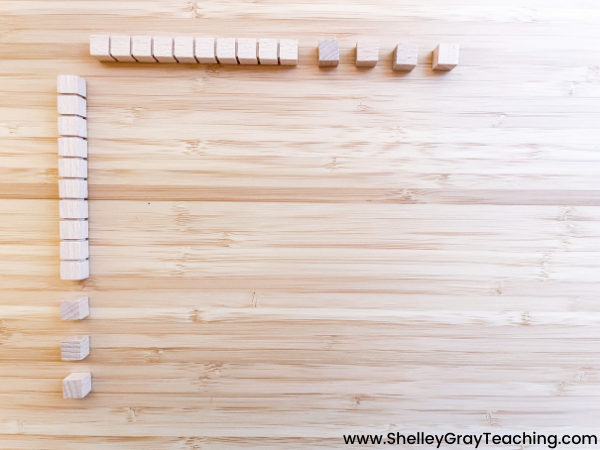Step 2: Fill the rectangle.

Now it’s time to figure out the area of the rectangle, which will become our product. First, we see that 10×10 is 100, so we place a hundred block in the rectangle.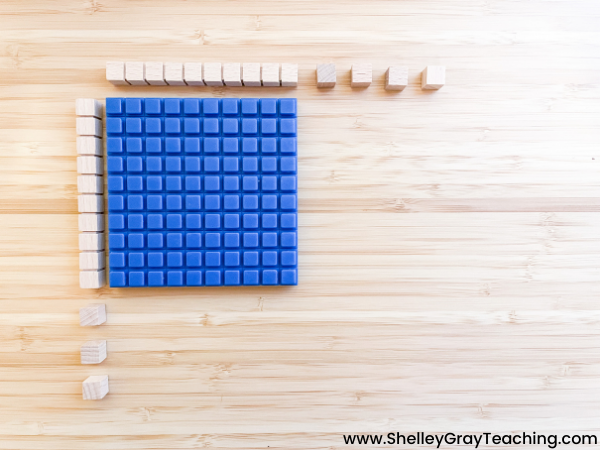Next, we see that 10×4=40, so we add 4 tens.Now let’s keep multiplying. 3×10=30, so we add 3 more tens.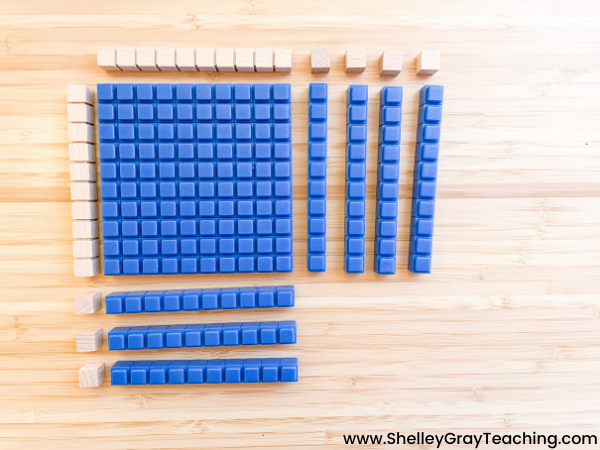Lastly, 3×4=12, so we add 12 ones.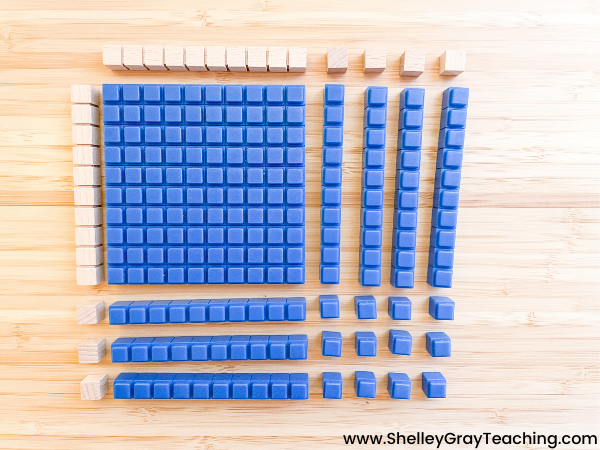Now we can see that the product of 13×14 is 182.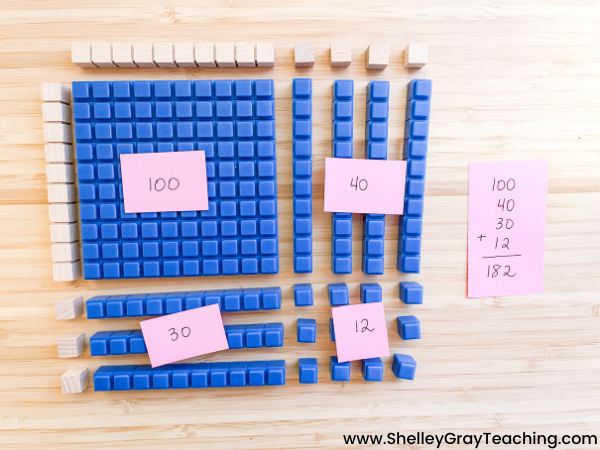Let’s see another example.

We can use the area model with basic multiplication facts, or with multi-digit multiplication. Here’s an example of how we can use the area model with a basic fact like 4×10.Extending Past Finding the Product

When we work with a strategy like the area model, it’s important to work with it in different ways. The focus should not always be on simply finding the product. Instead, we can work with finding unknowns or creating different equations that have the same product.

For example, we can show students a product (or area) and have them figure the factors (or side lengths).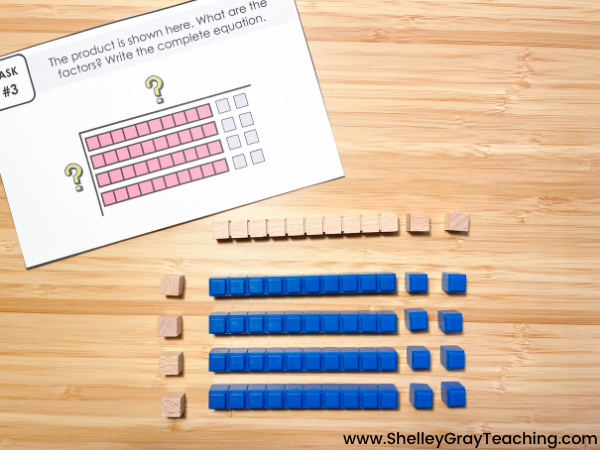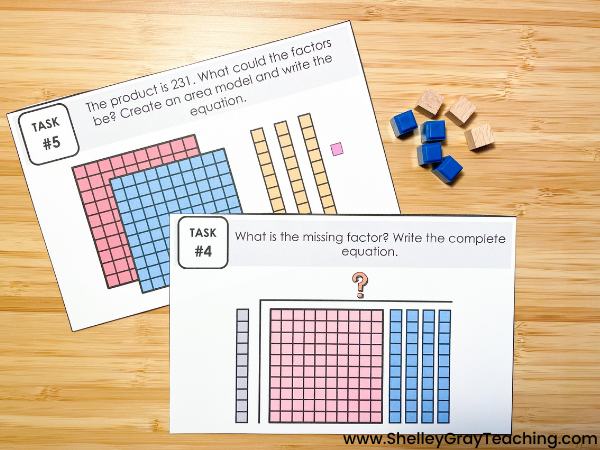We can also give students a variety of blocks, and have them create different area models using the same number of blocks. How does the product change? Why is this?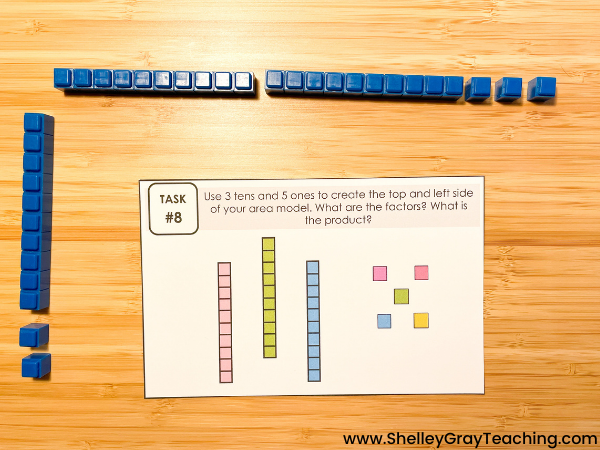This set of area model task cards will help students work with the area model in different ways, so they build the deep understanding that is necessary as they progress toward traditional long multiplication.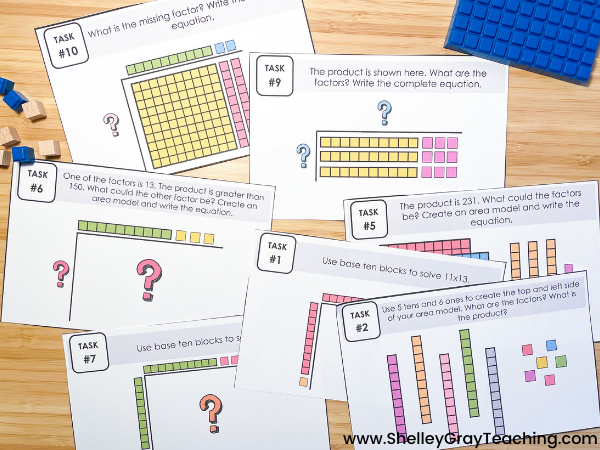Transitioning from Area Model With Base Tens to a Drawn Representation

Once students are comfortable with using base tens to create area models, THEN we can move into a drawn representation, as we talked about at the beginning of this article. It is essential that your students understand how the two different representations can be connected.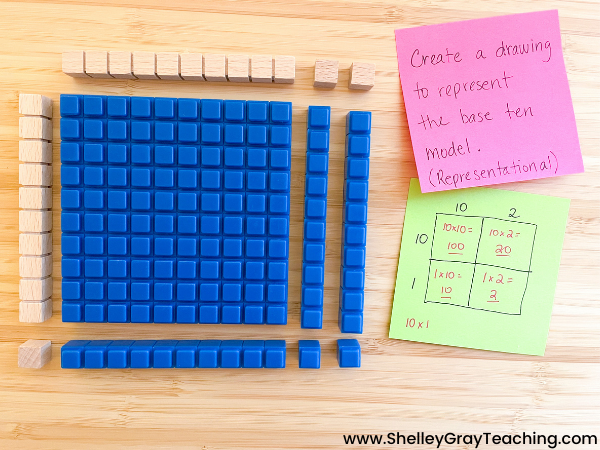What Comes After the Area Model?

The area model transitions beautifully into another strategy called partial products. This is essentially the same strategy, but we record our thinking differently. Partial products is set up like the traditional algorithm, but as you can see we are multiplying by place value, exactly as we do in the area model.If you plan to teach the traditional algorithm, it should come after students have used the area model and partial products extensively to build their overall understanding. Remember – math is not all about the answer. It is about the ability to think in flexible ways. Strategies like the area model allow our students flexibility in their thinking, and give students the opportunity to really SEE the math.

Resources to help you teach the area model with base ten blocks:This site uses Akismet to reduce spam. Learn how your comment data is processed.